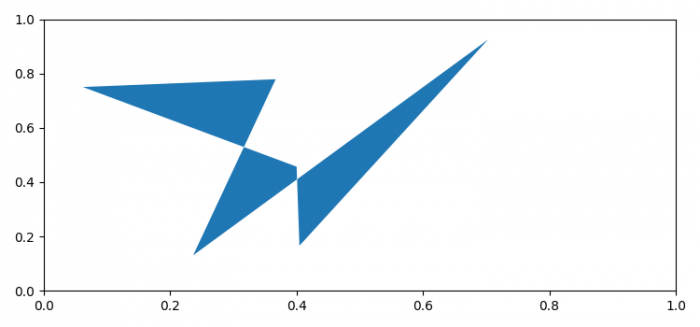# How to fill an area within a polygon in Python using matplotlib?

To fill an area within a polygon in Python using matplotlib, we can take the following steps −

## Steps

• Set the figure size and adjust the padding between and around the subplots.

• Create a figure and a set of subplots.

• Get an instance of a polygon.

• Get the generic collection of patches with iterable polygons.

• Add a 'collection' to the axes' collections; return the collection.

• To display the figure, use show() method.

## Example

import matplotlib.pyplot as plt
from matplotlib.collections import PatchCollection
from matplotlib.patches import Polygon
import numpy as np

plt.rcParams["figure.figsize"] = [7.50, 3.50]
plt.rcParams["figure.autolayout"] = True

fig, ax = plt.subplots(1)

polygon = Polygon(np.random.rand(6, 2), closed=True, alpha=1)

collection = PatchCollection([polygon])

plt.show()